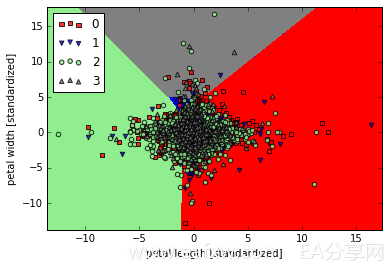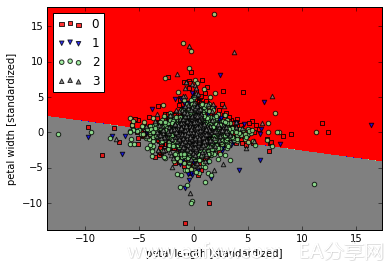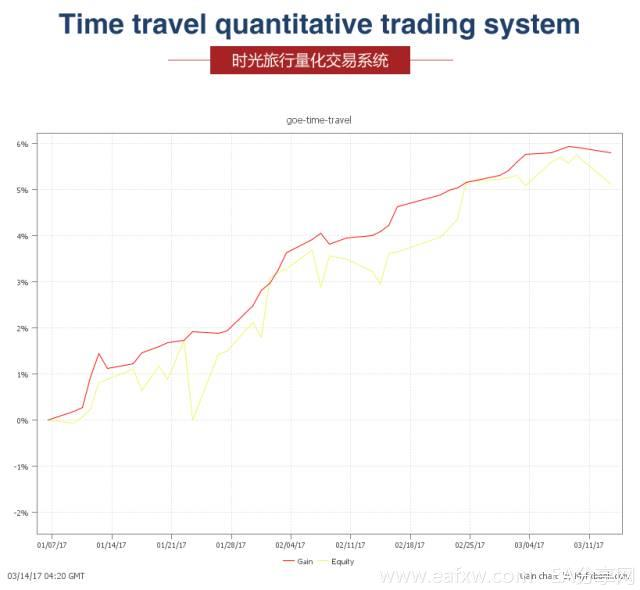1. 首先，把需要处理的数据准备好，

 前三根k线涨幅 当前k线涨幅 下一根大跌(0) /跌(1)/涨(2) /大涨(3) 10.36787091 14.01653763 1 0.56490792 -10.0514786 1 6.024039683 7.334618459 1 14.01653763 -4.792107118 1 -10.0514786 14.47722188 2 7.334618459 31.2593872 3 -4.792107118 11.13596167 0

Mt4中用脚本处理好数据，再写入csv里，作为我们的训练数据。

2. 导入数据，进行处理，

import pandas as pd

import numpy as np

from sklearn.cross_validation import train_test_split

from sklearn.preprocessing import StandardScaler

#获取经过mt4处理的数据集

#数据x,y分类

X = data.iloc[:,[1,2]].values

y = data.iloc[:,].values

y = y.ravel()

X_train,X_test,y_train,y_test = train_test_split(X , y , test_size = 0.35, random_state = 0)

sc = StandardScaler()

sc.fit(X_train)

X_train_std = sc.transform(X_train)

X_test_std = sc.transform(X_test)

3. 用sklearn的感知机模型训练数据

from sklearn.linear_model import Perceptron

#迭代次数1000次，学习率0.3

ppn = Perceptron(n_iter = 1000, eta0 = 0.3, random_state = 0)

ppn.fit(X_train_std,y_train)

y_pred = ppn.predict(X_test_std)

print ‘Misclassified samples:%d’ % (y_test != y_pred).sum()

print ‘Accuracy:%.2f’% accuracy_score(y_test,y_pred)

Misclassified samples:2942

Accuracy:0.471. 用逻辑回归模型预测涨跌

from sklearn.linear_model import LogisticRegression

lr = LogisticRegression(C=1000.0 , random_state  =0)

lr.fit(X_train_std,y_train)

y_pred = lr.predict(X_test_std)

print ‘Misclassified samples:%d’ % (y_test != y_pred).sum()

print ‘Accuracy:%.2f’% accuracy_score(y_test,y_pred)

Misclassified samples:2852

Accuracy:0.49